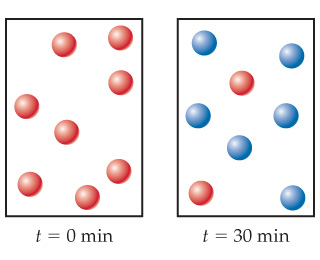# Problem: Given the following diagrams at t = 0 and t = 30, what is the half-life of the reaction if it follows first-order kinetics?

###### FREE Expert Solution

We are asked to find the half-life of the reaction from the diagram if it follows first-order kinetics.

The integrated rate law for a first-order reaction is as follows:

where:

[N]t = concentration of reactants at time t

k = decay constant

t = time

[N]0 = initial concentration###### Problem DetailsGiven the following diagrams at t = 0 and t = 30, what is the half-life of the reaction if it follows first-order kinetics?